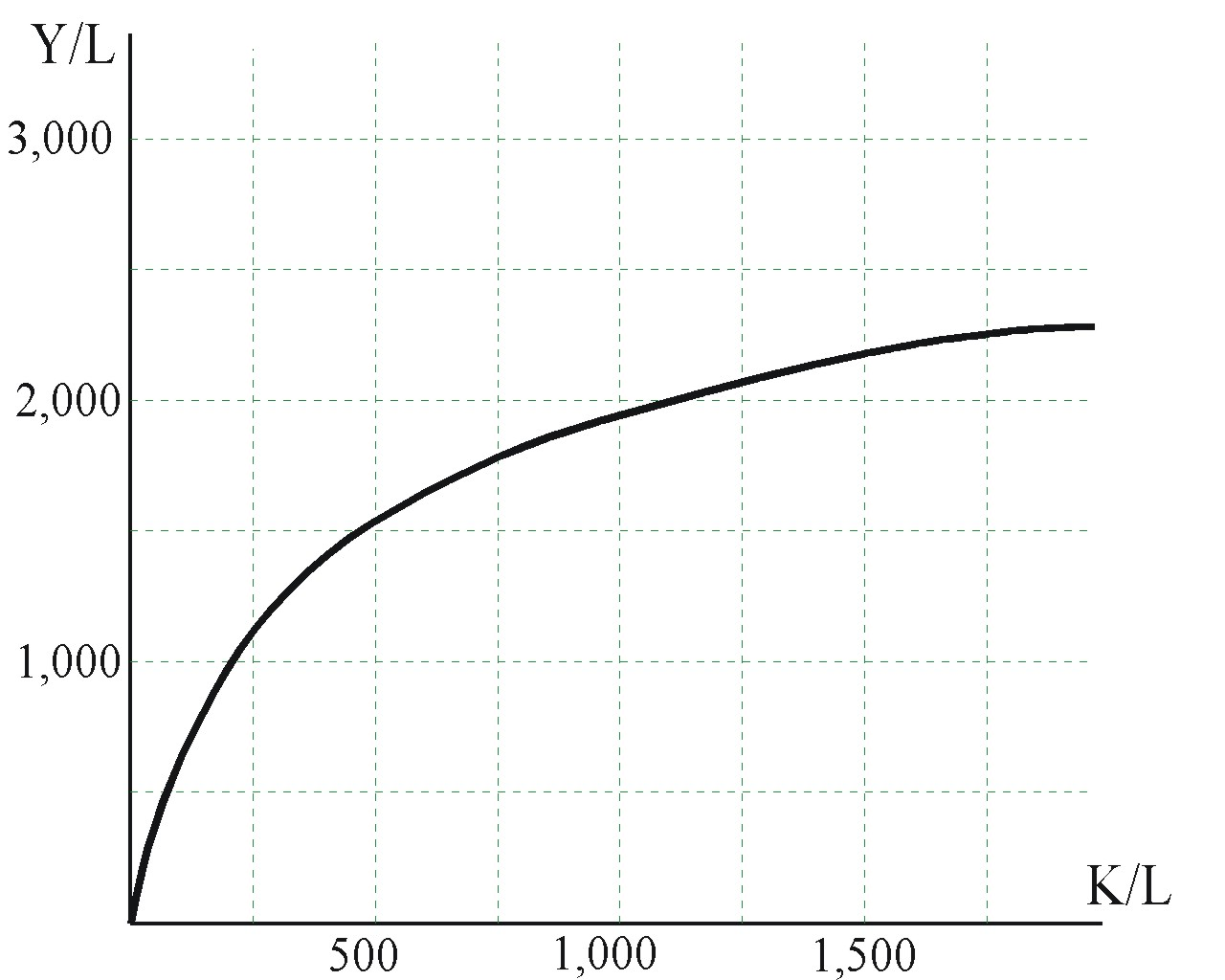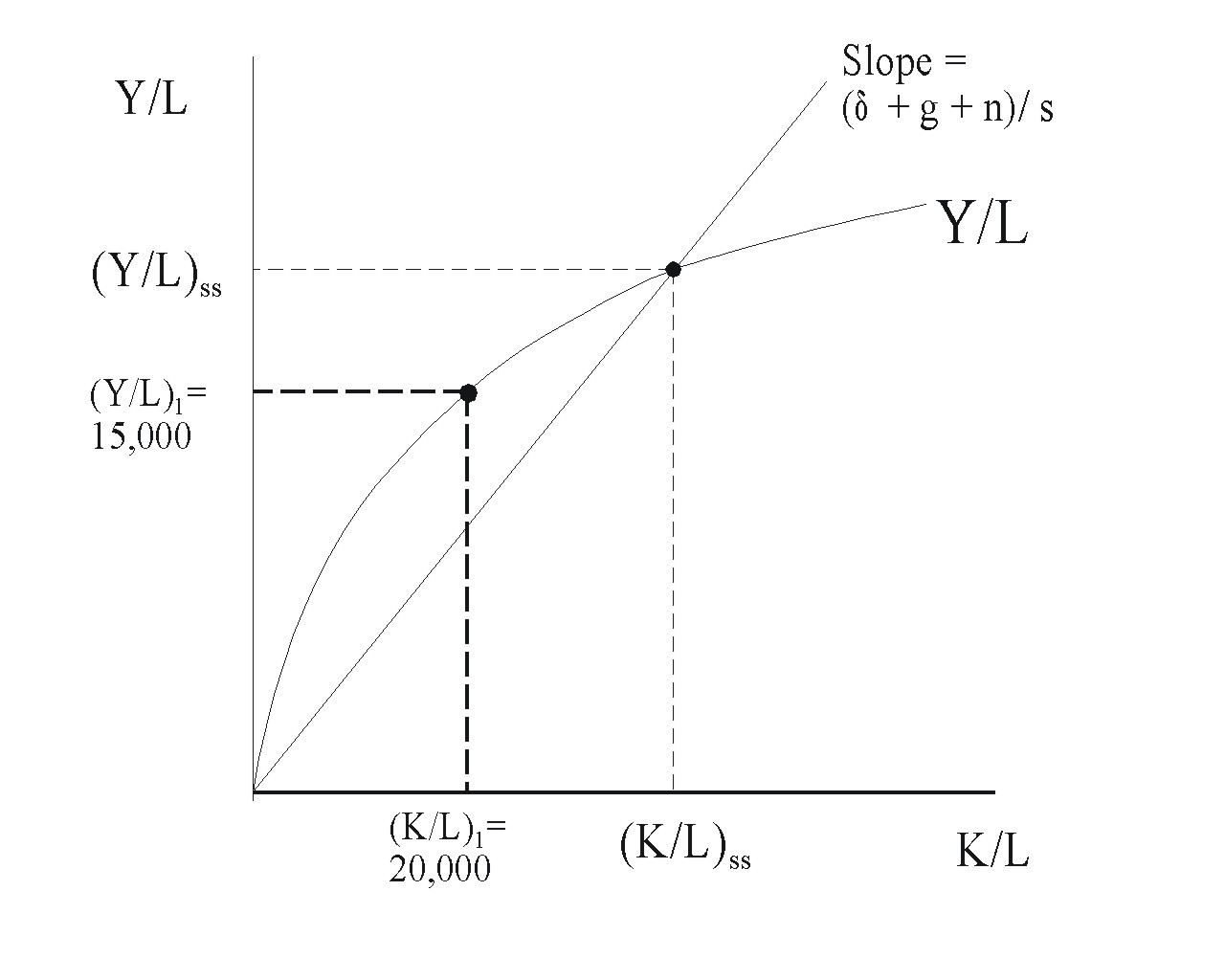Economics 100B Economic Analysis -- Macro University of California, Berkeley Spring 2019 Professor Martha Olney

## Old Quizzes

Some of the material on MT1 was covered in terms before Fall 2007 with an early-term 20-minute quiz.  Following are copies of questions from a quiz that Professor Olney used for Economics 100B previous times she taught the course (Fall 2004, Fall 2003, Spring 2003 -- fascinating to see what events were current at that time).   Answers to questions with "one right answer" are included. The answers immediately follow the question, in parantheses. If no answer is given, the question is an essay question.

QUIZ from Fall 2004 (10 points possible)

1.    (2 points) “Following Hurricanes Charley, Frances, Ivan, and Jeanne, homeowners in Florida hire workers to clean debris from their property.”  Where would this activity be recorded on the expenditure side of GDP accounting?  Your choices are C, I, G, GX, IM, and NR (not recorded).  Give a brief explanation of your answer.  (Answer:  C.  This is an expense by households for home maintenance.)

2.    (1 point) Within 1 percentage point, what is the current unemployment rate in the United States?  Has the unemployment rate increased, decreased, or stayed about the same since 2000?  (Answer:  Requires knowledge of current events.)

3.    (7 points)  Suppose the capital-to-labor ratio is 20,000, real GDP per worker is 2,000,  the personal saving rate is 20 percent, depreciation rate is 3 percent, labor efficiency is constant, and labor force is growing by 2 percent per year. Show all of your work, simplifying as far as possible without a calculator, or no points.  If you don’t attempt any simplifying, you won’t get as many points as someone who does simplify.

(A - 2 points)    <question deleted; not covered in current textbook>

(B - 1 point)    What is the definition of balanced-growth equilibrium? (no numbers needed here)  (Answer:  growth of K/L = growth of Y/L. Or, equivalently, K/Y is constant.)

(C - 2 points)    When this economy is in equilibrium, what is the value of the capital-output ratio?  (Answer:  K/Y = s / (n + g + depreciation rate) = 20 / (2 + 0 + 3) = 4.  Labor efficiency is constant, so g, the growth rate of labor efficiency, is 0.)

(D - 2 points)    Draw a graph that depicts this economy.   Show where the economy is currently located.  Label your axes and curves.  Label the current position with subscripts “1.”  (Answer:  graph has Y/L on vertical, K/L on horizontal.  Current K/L is to the right of the balanced-growth equilibrium.)

QUIZ from Fall 2003 (10 points possible)

1.    (4 points) “Following yet another announcement of security flaws in Microsoft Windows XP, Microsoft hires additional programmers to develop even more security patches.”  Where would this activity be recorded on the expenditure side of GDP accounting?  Your choices are C, I, G, GX, IM, and NR (not recorded).  Give a brief explanation of your answer.  (Answer:  NR.  Programmers are labor input to the production of the final product, Windows XP operating system.  Only expenditure for final products and for investment in physical capital is recorded on the expenditure side of GDP accounting.)

2.    (2 points) Within 1 percentage point, what is the current unemployment rate in the United States?  Has the unemployment rate increased, decreased, or stayed about the same over the last two years?

3.    (4 points)  Use the graph at the right for this question.   (on the original quiz, the grid lines showed more clearly)A    (1 point) When the capital to labor ratio doubles from 500 to 1,000, by how much does output per worker increase?  (Answer: Y/L increases by 500)

B    (1 point) When the capital to labor ratio increases from 1,000 to 1,500, by how much does output per worker increase?  (Answer:  Y/L increases by about 200)

C.    (2 points) Explain why the increase in the capital to labor ratio from 500 to 1,000 generates a larger increase in output per worker than does the increase in the capital to labor ratio from 1,000 to 1,500.  (Answer:  additional capital per worker generates additional output per worker -- output per worker goes up by 500, then by 200 --  but because as K/L increases, each worker is working with more and more capital, their ability to increase output is diminished with each increase in capital.  This is the essence of the concept of diminishing returns to investment.)

QUIZ from Spring 2003 (20 points possible)

1.    (4 points) “Heeding government warnings, American families buy plastic sheeting and duct tape to cover their windows in the event of terrorist attack.”  Where would this activity be recorded on the expenditure side of GDP accounting?  Your choices are C, I, G, GX, IM, and NR (not recorded).  Give a brief explanation of your answer.  (Answer:  C.  Households are purchasing final products, plastic sheeting & duct tape.)

2.    (2 points) Within 1 percentage point, what is the current unemployment rate in the United States?  Has the unemployment rate increased, decreased, or stayed about the same over the last two years?

3.    (10 points)  Suppose the capital-to-labor ratio is 20,000, real GDP per worker is 15,000,  the personal saving rate is 16 percent, depreciation rate is 5 percent, labor efficiency is constant, and labor force is growing by 3 percent per year. Show all of your work, simplifying as far as possible without a calculator, or no points.  If you don’t attempt any simplifying, you won’t get as many points as someone who does simplify.

(A - 3 points)    <question deleted; not covered in current textbook>
(B - 3 points)    When this economy is in equilibrium, what is the value of the capital-output ratio?  (Answer:  K/Y in equilibrium = 2)
(C - 4 points)    Draw a graph that depicts this economy.   Show where the economy is currently located.  Label your axes and curves.  Label the current position with subscripts “1.”4.     (4 points) Can increases in the saving rate provide increases forever in the standard of living?  Explain your answer. (Answer:  no.  There are limits to increasing the saving rate; it can be no more than 100%.  So at some point, when a maximum saving rate is reached, further increases in the saving rate are impossible, so further increases in the standard of living from this source are also impossible.)

Return to Economics 100B Home Page.